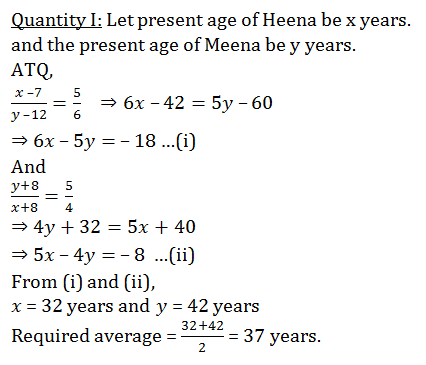# Quant Quiz for SBI PO PRE & LIC AAO 2019 (Day 48)|5th April 2019

## Quant Quiz for SBI PO PRE & LIC AAO 2019

Reasoning Quiz to improve your Quantitative Aptitude for SBI Po & SBI clerk exam Reasoning, IBPS PO Reasoning , IBPS Clerk Reasoning , IBPS RRB Reasoning, LIC AAO ,  and other competitive exams.

Q1. A 240 m long train takes 40 s longer to cross a platform twice its length than the time it takes to cross a pole at the same speed. What is the speed of the train?

A) 6 m/s

B) 24 m/s

C) 48 m/s

D) 12 m/s

E) None of these

Q2.  The distance between two stations A and B is 900 km. A train starts from A and moves towards B at an average speed of 30 km/hr. Another train starts from B, 20 minutes earlier than the train at A, and moves towards A at an average speed of 40 km/hr. How far from A will the two trains meet ?

A) 380 km

B) 320 km

C) 240 km

D) 330 km

E) None of these

Q3. Two trains were running in opposite directions at the speed of 48 kmph and 24 kmph respectively. If the faster train passed a man sitting in the slower train in 9.5 seconds, what is the length of the faster train?

A) 180m

B) 190m

C)  210m

D) 240m

E)  None of these

Q4. The length of train A is twice that of train B and speed of train A is half of that of train B. If train A crosses a man in 4 sec. then find how long will train B take to cross train A if they go in same direction.

A) 3s

B)  4s

C) 5s

D) 6s

E) None of these

Q5. Trains A and B are travelling at x km/hr and (x + 36) km/hr respectively. Train B crosses train A when running in the same direction inseconds. Find the sum of speed of both the trains if they pass each other in 9 seconds while running in opposite direction

A) 126 km/hr

B) 120 km/hr

C) 116 km/hr

D) 136 km

E)  115 km/hr

Q7.  Ratio of present age of A, B, C and D is 6 : 8 : 11 : 15 . Sum of their ages 4 years before was 64 years then what is the difference of present age of B and D.

A)  8 years

B) 14 years

C)  4 years

D) 22 years

E) None of these

Q8.  16 years hence, the age of Rashmi will be 10/13 th of the age of her friend Neha and 8 years ago, the ratio of their age (Rashmi : Neha) was 4 : 7. Present age of Neha is what percent more than the present age of Rashmi?

A) 50%

B) 40%

C) 60%

D) 60%

E) 60%

Q9.  Three friends P, Q and R have amount in the ratio of 5 : 6 : 8. If R givesof his amount to P then the difference of the amount left with R and the average of the amount of P and Q is Rs 560. Find the ratio of their initial amount after Rs 1000 is added to each of them.

A) 60 : 66 : 83

B) 67 : 72 : 81

C) 60 : 67 : 81

D) 60 : 67 : 81

E) 65 : 71 : 83

Q10.  The ratio of age of Heena 7 years ago to that of Meena 12 years ago was 5 : 6. And the ratio of age of Meena and Heena 8 years hence will be 5 : 4. Then find the average of their present age.

A) 37 years

B) 35 years

C) 32 years

D) 40 years

E) 45 years

## Solutions

Q1. Ans(D)Q2. Ans(A)Q3. Ans(B)Q4. Ans(D)Q5. Ans(A)Q7. Ans(B)

ATQ,
Let present age of A, B, C, D be 6x, 8x, 11x and 15x years respectively.
(6x – 4) + (8x – 4) + (11x – 4) + (15x – 4) = 64
40x = 80
x = 2
Difference of present age of B and D is
(15 – 8) × 2 = 14 years

Q8. Ans(A)Q9. Ans(C)Q10. Ans(A)### You Can Read This Also:

Best E-books for LIC AAO 2019 : Get PDF here

ambitiousbaba.com need your support to Grow

I challenge you will get Best Content in Our PDFs with Detail solutions and Latest Pattern

Memory Based Puzzle E-book | 2016-19 Exams Covered

Get PDF here

Caselet Data Interpretation 200 Questions

Get PDF here
Puzzle & Seating Arrangement E-Book for BANK PO MAINS (Vol-1)

Get PDF here

### ARITHMETIC DATA INTERPRETATION 2019 E-book

Get PDF here
The Banking Awareness 500 MCQs E-book| Bilingual (Hindi + English)

Get PDF here

High Level DATA INTERPRETATION Practice E-BOOK

Get PDF here

3